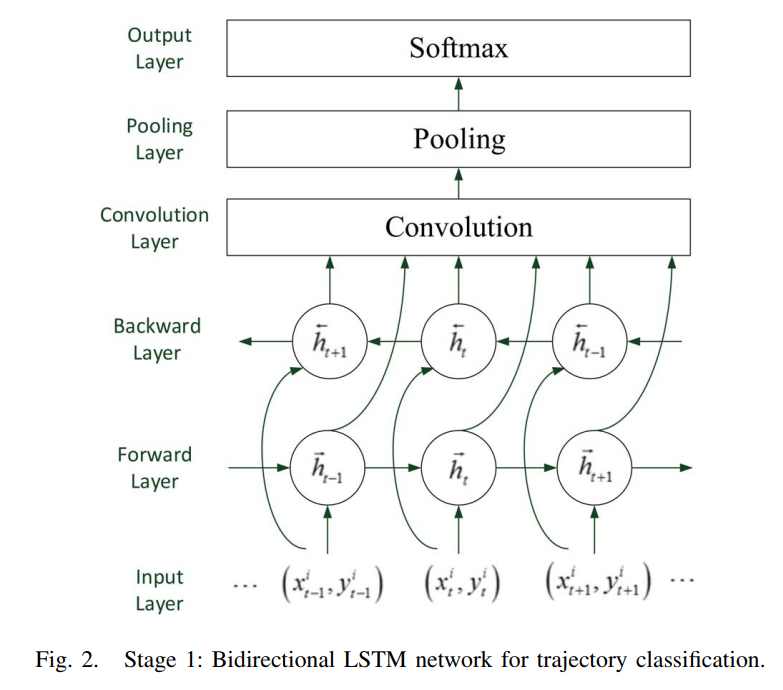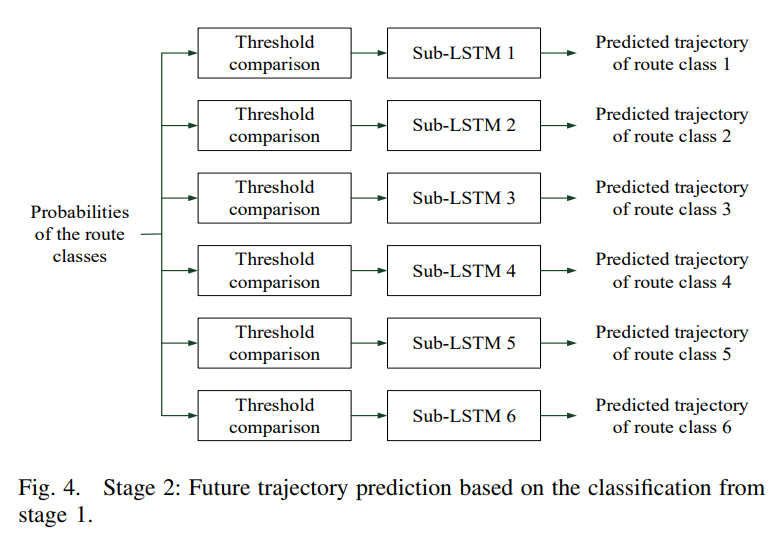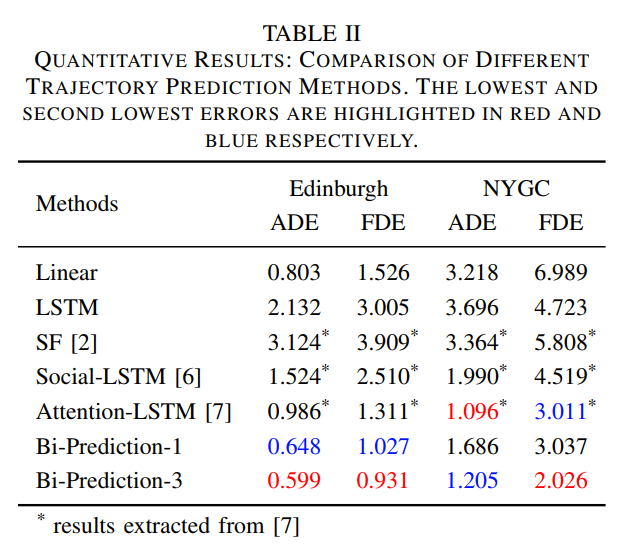@ArrowLLL 2018-02-27T02:44:16.000000Z 字数 5152 阅读 1631

# Study-Note : Pedestrian Trajectory Predition Based on Birdirectional LSTM Classfication

Study-Note OPTIMAL

## 中文理解

$X_i^{pred} = [(x_{obs+1}^i, y_{obs+1}^i), ... , (x_{pred}^i, y_{pred}^i)]$## Introduction

Existing research work on predestrian trajectory prediction can be divide into model based and Long Short Term Memory(LSTM) based methods.

Ideally, with multiple entry and exit points present in most scenes, atrajectory prediction method should predict multiple possible trajectories heading toward different destinations and each predicted trajectory should have a probability measure indiction how likely the trajectory would be taken.

we propose a novel two-stage trajectory prediction method to overcome the above shortcomings. After partitioning the scene into several regions, the first stage of our method is using bidirectional LSTM classification to predict a pedestrian's possible destination regions, with a short observed trajectory as input. The second stage is choosing differently trained LSTMs to predict the trajectory for each destination region.

This method is named Bi-Prediction, which has twi meanings: using bidirectional LSTM in trajectory prediction and having two stages in the whole process.

## Prosed Model

Problem

Given: Observed trajectories $X_i^{obs} = [(x_1^i, y_1^i), ..., (x_{obs}^i, y_{obs}^i)], \forall i$

Objective: Predict future trajectories $X_i^{pred} = [(x_{obs+1}^i, y_{obs+1}^i), ... , (x_{pred}^i, y_{pred}^i)], \forall i$

Our proposed two-stage Bi-Prediction method can learn the potential destinations in a scene and can generate multiple trajectory predictions.

first stage: to predict the destination candidates $D_i$ and the probability of choosing $D_j$.

second stage: to generate different sequences $X_{i,j}^{pred}$ based on these candidate destinations $D_j$.

### Stage 1: Bidirectional LSTM ClassficationTo deal with the complex movement patterns of pedestrians, the scene is first partioned into regions.

It is import to note that the route is not well-defined if the trajectory is very short. One way to overcome this is to design a special network that combines a recurrent neural network woth a convolution neural network whereby the former develops the "foreseeing" power and the letter takes charge of classification task to output multiple probabilities of different destination regions.

For the bidirectional LSTM network architecture :

Specifically, $\overleftarrow h_t, \overrightarrow{W}, \overleftarrow{h}_t$ and $\overleftarrow{W}$ are the hidden states and weight matrices of the forward and backward layers respectively.

### Stage 2: Prediction with Classification

To predict future trajectories corresponding to different route classes.

When it comes to future trajectory prediction, existing LSTM based methods which use the one-LSTM-one-pedestrian policy would suffice, which make both the training process and the predicting process very computationally expensive; one LSTM for predicting the trajectories of all pedestrians, the network would not be able to learn all kinds of pedestrian movement. As a trade-off, our method uses one sub-LSTM for each route class.If the probability of a route class is larger than a pre-defined threshold $\tau$, the system automatically selects the corresponding trained sub LSTMs to output the predicted trajectories for each pedestrian.

As for each sub-LSTM, we use a general encoder-decoder network where the LSTM encoder layer receives an observed trajectory as input and generates a hidden state sequence. From the hidden state sequence, the LSTM decoder layer can output a predicted trajectory. The input size and output size of the sub-LSTMs are determined by the length of the observed trajectories and predicting trajectories respectively.

### Implementation Details

• the forward and backward LSTM layers have a fixed hidden state dimension of 128.
• We use a one dimension convilution layer follwoed by one dimension max pooling layer. The size of the max pooling windows is 4.
• the hidden states is also 128-dimensional for each sub-LSTM in stage 2.
• Model is built using Python on Keras with a Tensorflow backend.
• The learning rate is set to 0.003.
• Use 20-frame observed trajectories to train each sub-LSTM and predict trajectories of 20 frames long. $T_{obs} = 20 and T_{pred} = 40$
• The pre-defined threshold $\tau$ is set to 0.01.

## Experiments

Datasets:

1. New York Grand Central dataset(NYGC)
2. Edinburgh Informatics Forum(EIF)

Metrics:

• Final displacement error(FDE)

Compare:

two baseline methods and 3 state-of-art pedestrian trajectory prediction methods with which we compare our method are:• Linear Prediction
• LSTM
• Social Force(SF)
• Social-LSTM
• Attention-LSTM
• Bi-Prediction-1
• Bi-Prediction-3• 私有
• 公开
• 删除